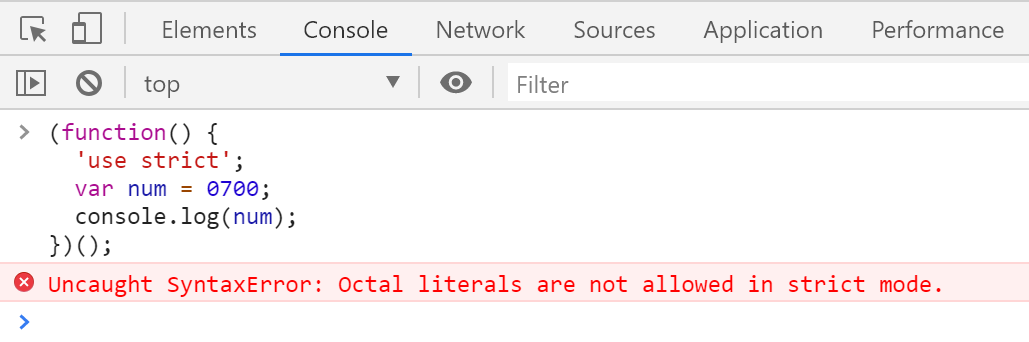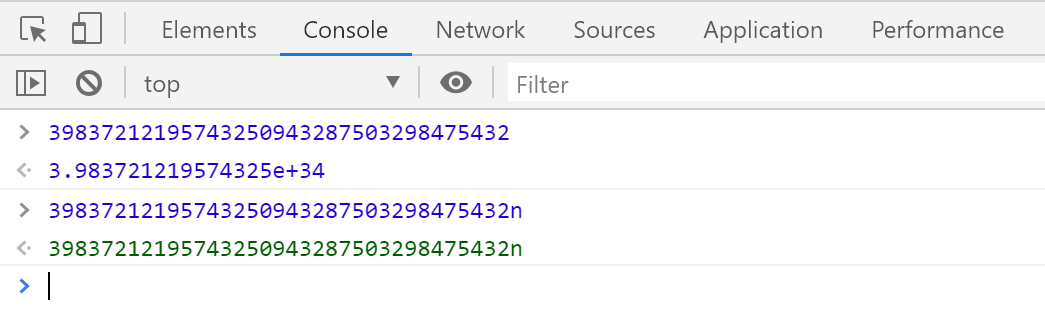前端工程研究：關於 JavaScript 中 Number 型別的常見地雷與建議作法 | The Will Will Web# The Will Will Web

### 記載著 Will 在網路世界的學習心得與技術分享### 數值的表示法

• 16 進制

``````let num = 0xFFF;
``````
• 10 進制

``````let num = 100;
``````
• 8 進制

``````let num = 0700;
``````

這裡有點需要注意，從 ES2015 開始，在嚴格模式下的 JS 就不允許使用這種 8 進制數值表示法 (Octal literals)。

``````(function() {
'use strict';
let num = 0700;
console.log(num);
})();
``````如果要的話，必須改用 ES2015 全新的 8 進制表示法，必須以 `0o``0O` 為前綴：

``````(function() {
'use strict';
let num = 0o700;
console.log(num);
})();
``````
• 2 進制

使用 2 進制必須以 `0b``0B` 為前綴，此語法是 ES2015 才新增的表示法：

``````let num = 0b1111;
``````
• 科學記號表示法 (Scientific notation)

以下這段數值等於 10 乘以 10 的 5 次方：

``````let num = 10e5;
``````

以下這段數值等於 3.1415 乘以 10 的 -3 次方：

``````let num = 3.1415e-3;
``````
• 無窮大 (Infinity)

正無窮大有兩種表示法，一種是瀏覽器內建的 `Infinity` 全域變數。另一種則是 ECMAScript 定義的 `Number.POSITIVE_INFINITY` 也可以直接使用：

``````let num = Infinity;
let inf = Number.POSITIVE_INFINITY;
let sho = 1/0; // 更短的正無窮大寫法
``````

負無窮大也有兩種表示法：

``````let num = -Infinity;
let inf = Number.NEGATIVE_INFINITY;
let sho = -1/0; // 更短的負無窮大寫法
``````

在瀏覽器中，這裡的 `Infinity` 其實是 `window` 根物件下的一個屬性，可以被視為全域變數，所以你也可以寫成：

``````let num = window.Infinity;
``````

在 ES2015 版本裡，你可以透過一個 Number 型別內建的 `Number.isFinite()` 函式來判斷該數值物件是否為有限的數值

``````Number.isFinite(46872394293) // true
``````
• NaN (Not a Number)

這是一個相當特別的「數值」物件，從名稱上來看 `NaN` 就是 `Not a Number` 的縮寫，但是透過 `typeof(NaN)` 你又會得到 `"number"` 的結果，代表這是一個 `number` 型別，但本身不是一個數值，你無法對 `NaN` 物件做任何四則運算！

``````let num = NaN;
``````

你要判斷一個變數是否為 `NaN`，絕對不能用以下判斷式：

``````let num = NaN;
num === NaN // false
``````

要判斷某數是否為 `NaN` 只能透過以下方法：

``````let num = NaN;
window.isNaN(num); // true

// 由於 isNaN 是 window 下的一個屬性，因此可以視為全域的存在，因此可以簡化成以下寫法
isNaN(num); // true
``````

在 ES2015 版本裡，你可以透過一個 Number 型別內建的 `Number.isNaN()` 函式來判斷該數值物件是否為NaN

``````Number.isNaN(num); // true
``````

``````1_000_000_000           // Ah, so a billion
101_475_938.38          // And this is hundreds of millions

let fee = 123_00;       // \$123 (12300 cents, apparently)
let fee = 12_300;       // \$12,300 (woah, that fee!)
let amount = 12345_00;  // 12,345 (1234500 cents, apparently)
let amount = 123_4500;  // 123.45 (4-fixed financial)
let amount = 1_234_500; // 1,234,500
``````

### 數值型別轉換

• 字串轉數字

你可以透過 `Number` 函式來轉換字串數值

``````Number('3.14')   // 3.14
Number('100')    // 100
Number(' ')      // 0
Number('')       // 0
Number('a123')   // NaN
Number('1,000')  // NaN
``````

也可以透過 `+` (加號) 來轉換數值，這算是一個相當常見的數值轉換技巧：

``````+'3.14'   // 3.14
+'100'    // 100
+' '      // 0
+''       // 0
+'a123'   // NaN
+'1,000'  // NaN
``````

另一種常見字串數值的方式，則是使用 parseIntparseFloat 函式來解析字串。

這裡你必須特別注意這兩個函式的定義：

1. `parseInt(str [, radix])`

第 2 個參數 `radix` (基數) 代表第 1 個參數的內容代表哪種進制，預設值為 `10` 進制。你可以設定 2 ~ 36 的數字都可以，最大值不能超過 `36` 進制。

所謂 36 進制，代表你可以用 `0-9``A-Z` 等 36 個字元當成數值表示。

2. `parseFloat(str)`

這個函式只能固定轉換十進制的實數。

這兩個函式在傳入第 1 個參數時，都要務必確認此參數只能是 字串 (`string`) 型態，千萬不要傳入 非字串 的物件進來，否則你就會得到以下詭異的結果：

``````parseInt(0.1) // 0
parseInt(0.01) // 0
parseInt(0.001) // 0
parseInt(0.0001) // 0
parseInt(0.00001) // 0
parseInt(0.000001) // 0
parseInt(0.0000001) // 1
``````

為什麼數值小到一定程度，就會導致 `parseInt` 的結果發生異常呢？那是因為從 `0.0000001` 開始，該數值會被轉成科學記號表示法，實際上會變成 `1e-7`，而 `parseInt` 需要將第一個參數轉為字串，因此你傳入的 字串 其實是 `"1e-7"`，而對 `parseInt` 來說，他會從字串最左邊開始解析每個認得的數字符號，遇到無法解析的字元就會自動忽略所有剩餘的內容。以這個例子來說，`parseInt` 指任何 `1e-7``1` 而已，因為預設 `radix``10` 進制，因此 `e` 這個字元是看不懂的，所以最終結果為 `1`，非常意外吧！ 😅

如果要解析複雜的數值字串，可以考慮採用 Numeral.js 函式庫。

• 布林轉數值

使用 `Number(false)` 會將布林值 `false` 轉為數字為 `0`

使用 `Number(true)` 會將布林值 `true` 轉為數字為 `1`

因此，以下運算式就不足為奇，不是嗎？ 😅

``````1 < 2 < 3 // true
``````
``````3 > 2 > 1 // false
``````
• 日期轉數值

在 JavaScript 中的 Date 型別用來指向某一個時間點，但事實上在骨子裡儲存的其實是 `number` 型態，你可以透過 `.valueOf()` 方法取得內部的數值：

``````new Date(2020, 1, 20, 0, 0, 0).valueOf() // 1582128000000
``````

也可以透過 `+` (加號) 來轉型：

``````+new Date(2020, 1, 20, 0, 0, 0) // 1582128000000
``````

或是透過 `Number()` 來轉型：

``````Number(new Date(2020, 1, 20, 0, 0, 0)) // 1582128000000
``````
• 數字轉字串 (預設為 10 進制)

``````let num = 566;
num.toString(); // "566"
``````
• 數字轉字串 (指定基數)

將數值轉為 16 進制的字串

``````let num = 65535;
num.toString(16); // "ffff"
``````

將數值轉為 36 進制的字串

``````let num = 65535;
num.toString(36); // "1ekf"
``````

### 整數運算的地雷

JavaScript 採用 IEEE-754 Floating Point 規定的浮點數計算所有數值，但是有計算上的精準度問題，任何整數數值超過 `2^53-1` (`9007199254740991`) 就會開始產生誤差，這是一個非常地雷的數值邊界，必須特別注意。

``````Number.MAX_SAFE_INTEGER == 2**53-1 // true
``````

``````Number.MAX_SAFE_INTEGER+0 // 9007199254740991
Number.MAX_SAFE_INTEGER+1 // 9007199254740992
Number.MAX_SAFE_INTEGER+2 // 9007199254740992
Number.MAX_SAFE_INTEGER+3 // 9007199254740994
Number.MAX_SAFE_INTEGER+4 // 9007199254740996
Number.MAX_SAFE_INTEGER+5 // 9007199254740996
Number.MAX_SAFE_INTEGER+6 // 9007199254740996
Number.MAX_SAFE_INTEGER+7 // 9007199254740998
Number.MAX_SAFE_INTEGER+8 // 9007199254741000
Number.MAX_SAFE_INTEGER+9 // 9007199254741000
``````

``````999999999999999         // 999999999999999
9999999999999999        // 10000000000000000
10000000000000000 + 1.1 // 10000000000000002

9999999999999999999999 + 100 === 9999999999999999999999 // true
``````

``````Number.isSafeInteger(46872394293) // true
Number.isSafeInteger(-4362794324) // true
``````

``````Number.isInteger(4687239); // true
Number.isInteger(4687239.4293); // false
``````

### 超大整數 (BigInt)

``````let num = 39837212195743250943287503298475432n
typeof(num); // bigint
``````

``````50000n/2n === 25000n
``````

``````2n**50000n
``````

``````BigInt('39837212195743250943287503298475432')
``````

``````39837212195743250943287503298475432  // 3.983721219574325e+34
39837212195743250943287503298475432n // 39837212195743250943287503298475432n
````````````BigInt(999999999999999999999999) // 999999999999999983222784n
BigInt(1.5)                      // RangeError: 不能有小數
BigInt('1.5')                    // SyntaxError: 語法錯誤
1 + 1n                           // TypeError: 不能混用型別
new BigInt(123)                  // TypeError: 不能用 new 建立物件
``````

### 浮點數運算的地雷

``````(1).toString(2)        // 1
(0.5).toString(2)      // 0.1
(0.25).toString(2)     // 0.01
(0.125).toString(2)    // 0.001
(0.0625).toString(2)   // 0.0001
(0.03125).toString(2)  // 0.00001
``````

``````(0.1).toString(2) // "0.0001100110011001100110011001100110011001100110011001101"
``````

``````(0.2).toString(2) // "0.001100110011001100110011001100110011001100110011001101"
``````

``````0.1 + 0.2 != 0.3
0.2 + 0.4 != 0.6
0.3 + 0.6 != 0.9
0.4 + 0.8 != 1.2
``````

``````0.1 + 0.2 // 0.30000000000000004
0.2 + 0.4 // 0.6000000000000001
0.3 + 0.6 // 0.8999999999999999
0.4 + 0.8 // 1.2000000000000002
``````

``````Math.ceil(0.1*0.2*100)   // 3
Math.ceil(0.1*0.2*1000)  // 21
Math.ceil(0.1*0.2*10000) // 201
``````

### 數值型別的最大值與最小值

``````Number.MAX_VALUE == 1.7976931348623157e+308
Number.MIN_VALUE == 5e-324
``````

``````Number.MIN_VALUE > 0 // true
``````

``````Math.max(2, 4, 6, 8); // 8
Math.min(1, 3, 5, 7); // 1
``````

``````Math.max() > Math.min(); // -> false
Math.min() > Math.max(); // -> true
``````

### 其他技巧

• 自動取整數

``````parseInt(2.99)
``````

請注意：由於 `parseInt` 的第一個參數必須傳入字串型別的物件，若傳入的是一個 `number` 的話，預設會自動先轉成字串，才會開始進行解析。

``````~~2.99
``````

因為 `~` 是一種位元運算子，所有位元運算子運算元皆會被轉換成二補數系統下的帶號32位元整數。所以，如果你使用 `~~` 技巧來取整數，須注意數值不可大於等於 2 的 31 次方 (`2147483648`)，否則會發生溢位，而導致結果為負數！(詳見 MDN位元運算子 說明)

• 自動取小數幾位

``````parseInt(2.99 * 10) / 10
``````
``````+(2.999).toFixed(3)
``````
• 四捨五入 (負數超過 0.5 才會進位)

``````Math.round(2.5) == 3
Math.round(-2.5) == -2
Math.round(-2.51) == -3
``````
• 無條件捨去 (小於自己的最大整數)

``````Math.floor(2.99) == 2
``````
• 無條件進位 (大於自己的最小整數)

``````Math.ceil(2.11) == 3
``````

### 結論

• ``````numeral('10,000.12').value()  // 10000.12
numeral('\$10,000.00').value() // 10000

numeral(974)          // 974
numeral(0.12345)      // 0.12345
numeral('10,000.12')  // 10000.12
numeral('23rd')       // 23
numeral('\$10,000.00') // 10000
numeral('100B')       // 100
numeral('3.467TB')    // 3467000000000
numeral('-76%')       // -0.76
numeral('2:23:57')    // NaN
``````
• ``````+Decimal(0.1).add(Decimal(0.2))          // 0.3

x = new Decimal(9)                       // '9'
y = new Decimal(x)                       // '9'

new Decimal('5032485723458348569331745.33434346346912144534543')
new Decimal('4.321e+4')                  // '43210'
new Decimal('-735.0918e-430')            // '-7.350918e-428'
new Decimal('5.6700000')                 // '5.67'
new Decimal(Infinity)                    // 'Infinity'
new Decimal(NaN)                         // 'NaN'
new Decimal('.5')                        // '0.5'
new Decimal('-0b10110100.1')             // '-180.5'
new Decimal('0xff.8')                    // '255.5'

new Decimal(0.046875)                    // '0.046875'
new Decimal('0.046875000000')            // '0.046875'

new Decimal(4.6875e-2)                   // '0.046875'
new Decimal('468.75e-4')                 // '0.046875'

new Decimal('0b0.000011')                // '0.046875'
new Decimal('0o0.03')                    // '0.046875'
new Decimal('0x0.0c')                    // '0.046875'

new Decimal('0b1.1p-5')                  // '0.046875'
new Decimal('0o1.4p-5')                  // '0.046875'
new Decimal('0x1.8p-5')                  // '0.046875'
``````
• ``````new BigNumber(43210)                       // '43210'
new BigNumber('4.321e+4')                  // '43210'
new BigNumber('-735.0918e-430')            // '-7.350918e-428'
new BigNumber('123412421.234324', 5)       // '607236.557696'
new BigNumber('-Infinity')                 // '-Infinity'

new BigNumber(NaN)                         // 'NaN'
new BigNumber(-0)                          // '0'
new BigNumber('.5')                        // '0.5'
new BigNumber('+2')                        // '2'

new BigNumber(-10110100.1, 2)              // '-180.5'
new BigNumber('-0b10110100.1')             // '-180.5'
new BigNumber('ff.8', 16)                  // '255.5'
new BigNumber('0xff.8')                    // '255.5'

BigNumber.config({ DECIMAL_PLACES: 5 })
new BigNumber(1.23456789)                  // '1.23456789'
new BigNumber(1.23456789, 10)              // '1.23457'

new BigNumber('5032485723458348569331745.33434346346912144534543')
new BigNumber('4.321e10000000')

new BigNumber('.1*')                       // 'NaN'
new BigNumber('blurgh')                    // 'NaN'
new BigNumber(9, 2)                        // 'NaN'
``````
• ``````x = new Big(9)                       // '9'
y = new Big(x)                       // '9'
Big(435.345)                         // 'new' is optional
new Big('5032485723458348569331745.33434346346912144534543')
new Big('4.321e+4')                  // '43210'
new Big('-735.0918e-430')            // '-7.350918e-428'
``````
• What is the difference between big.js, bignumber.js and decimal.js?